- JoNova - http://joannenova.com.au -

New Science 2: The Conventional Basic Climate Model — the engine of “certain” warming

Posted By David Evans On September 23, 2015 @ 6:41 pm In Global Warming | Comments Disabled

This is the most uncontroversial post ever put on this blog — it’s everything the IPCC would agree with and the key to their unshakable confidence.

This post is for the independent thinkers, the brains that want to know exactly where the famous, core, 1.2 °C figure comes from. That’s the number of degrees that a doubling of CO2 would bring, and it’s a figure that underlies decades of research and the figure that the big models are built around. Here, as far as we know, is the simplest, accurate reference to that reasoning and their maths. We have always assumed the 1.2 °C figure is correct, and focused on questioning the feedbacks that are assumed to drive that base figure up to  3°C (or 6°C or molten-Venus-here-we-come!)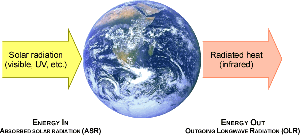We are not criticizing the estimate here, but this is so key and central to the whole climate-clean-green industry, and the models, it has to be laid out. This is the source of “implacable confidence” among the leading thinkers of establishment climate science. It is long past time that skeptical scientists put these details — warts and all — out in public. Dr David Evans is laying out the foundation for a complete and systematic analysis. The heaviest stuff is here and in the next post. The exciting stuff starts after that. For those who want to follow the unfolding science pay attention to the acronym table (and diagrams) below. The notations like ASR and OLR are the language we need to speak.

For those who are not number-heads, stay tuned, I will be spacing other news and stories among the serious science posts, often doing more than one post a day. It’s going to be very busy as I feed in the cutting edge research to the usual mix of politics, citizen science, quirky news and satire.  –  Jo

# 2. The Conventional Basic Climate Model — Simple

Dr David Evans, 23 September 2015, David Evans’ Basic Climate Models Home, Intro, Next, Nomenclature.

The conventional basic climate model is moderately complicated when all the bits and pieces are included, so we are going to present it over two blog posts. In this post we’ll just consider the simplest case, direct warming, where the only input is the change in carbon dioxide level, and there are no feedbacks.

### Introduction

For those not accustomed to mathematical modelling, this post might seem complicated. To others it may appear rudimentary and insubstantial—“surely there is more to it”. It is ruthlessly logical, squeezing as much information as possible from clues and relationships, but be aware as you read through it of the vast number of climate factors that are ignored.

I’ve tried to make it easier to follow by supplementing many equations with explanations, aiming for a broader audience than just STEM people. With enough diligence and patience (yes, it does take time to think about it), most readers will be able to at least get the general idea.

In any case, it might satisfy your curiosity about how models are made and why some people believe in the carbon dioxide theory despite all the contrary evidence.

There are “partial derivatives” in what follows. They can sound intimidating if you aren’t familiar with them, but a partial derivative is just how quickly one thing changes as another changes, if all else is held constant. For example, how the  height above sea level changes as you move north is a partial derivative — it is the slope of the surface you are on (such as a hill) as you move north.  Partial derivatives are intrinsic to the model, so there is no avoiding them.

The conventional basic climate model is partially described by two foremost theorists, Isaac Held and Brian Soden, in their paper of 2000 , and more completely on pages 163–165 of the “gold standard” of climate textbooks, Raymond Pierrehumbert’s Principles of Planetary Climate  (recommended if you want to know establishment climate science). We get the parameter values from the IPCC’s latest assessment report from 2013, AR5 .

All the models in this blog series are for the passage of Earth from one steady state to another. If the Earth is in “steady state”, everything is more or less in balance and stable, and its statistical properties are constant — it’s not in a transient state. This is not quite the same concept as “equilibrium”, because energy is flowing into and out of the system rather than just back and forth between different parts of the system.

We employ standard notation: the value of a variable (e.g. X) in the initial steady-state has a “0” subscript (e.g. X0), while the change from the initial to the final steady state is prefixed with a delta “Δ” (e.g. ΔX). The variables for the final steady state have no subscript (e.g. X). Thus ΔX equals XX0.

In steady state, the outgoing long-wave radiation (OLR, the heat or energy radiated to space, denoted by R) is equal to the absorbed solar radiation (ASR, the unreflected portion of the radiation from the Sun that is incident upon the Earth, denoted by A); both the ASR and OLR are about 239 W/m2. This is known as “energy balance” or “radiation balance”, and follows by the conservation of energy applied to the planet: energy in equals energy out. Thus, between two steady states, the change in ASR equals the change in OLR: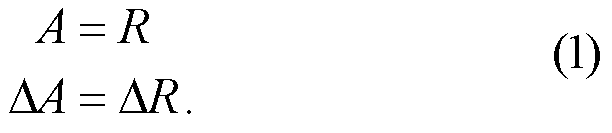Figure 1: ASR in, OLR out. In and between steady states, ASR = OLR.

### Acronyms and Symbols

 ASR A Absorbed solar radiation (energy from the Sun that is not reflected) CO2 C Carbon dioxide ECS Equilibrium climate sensitivity (the increase in TS when CO2 doubles) EDA Externally-driven albedo (albedo independent of surface warming) L Base-2 logarithm of the CO2 concentration C OLR R Outgoing longwave radiation (heat/energy from Earth to space) TOA Top of the atmosphere TSI S Total solar irradiance (energy/heat from the Sun, incident on Earth) TS Surface temperature (global average air temperature at the surface) WVEL Water vapor emission layer (average height of optical top of water vapor)

Table 1: Commonly used acronyms and symbols in this blog series (also see the Nomenclature pdf, on the author line under the title).

### The Set-Up: Only CO2 and Temperature Can Change

Consider the hypothetical situation where only the CO2 concentration and the temperature can change, with everything else about the climate held constant.

The CO2 concentration is represented by C. The amount of OLR blocked by rising CO2 rises logarithmically with C and is thus represented by its base-2 logarithm L: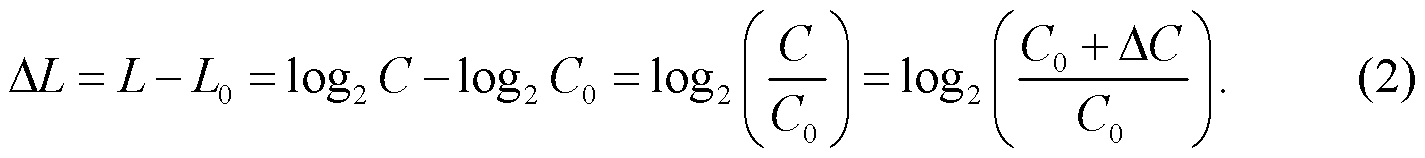The mean tropospheric temperatures are assumed to all change uniformly — because the lapse rate is assumed to remain constant, like everything else except CO2 and temperature. Thus the change in any tropospheric temperature is equal to ΔTS, the surface warming, where TS is the mean surface temperature. For example, if the surface warms by 1 °C then the whole troposphere warms by 1 °C and ΔTS equals 1 °C.

### How Much Does the OLR Change?

The crucial question: if CO2 and surface temperature each change, how much does the OLR change?

Suppose that the logarithm of the CO2 concentration increases by an incremental amount dL, and the surface warms by an incremental amount dTS (the prefix “d” means an incrementally small increase in the variable that follows, a calculus notation). What is the corresponding change in the OLR R (dR, also incremental)?

The OLR depends only on the surface temperature and the CO2 concentration, because everything else is held constant, so it is a function of just the two variables TS and L. Treating the arguments of R as two independent variables,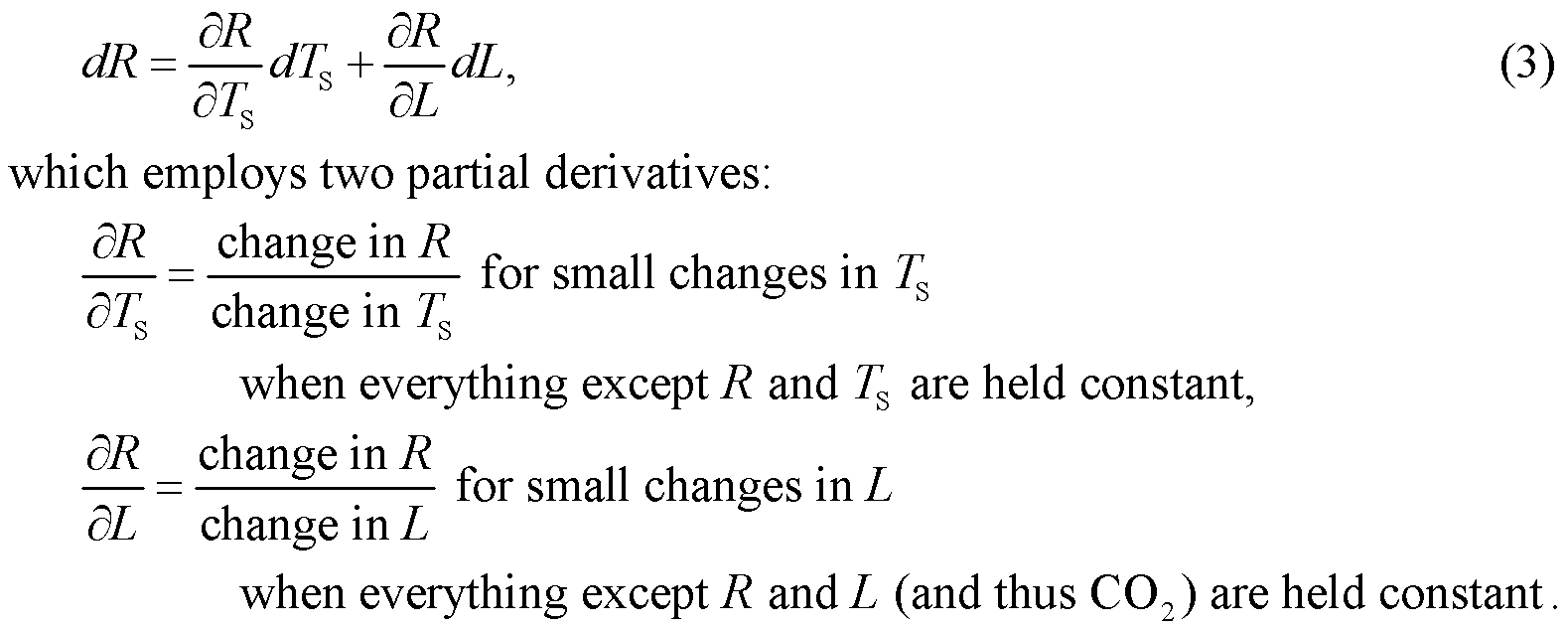This is an application of a well-known rule of calculus for partial derivatives. The first partial derivative is the ratio of change in OLR to change in surface temperature if only the OLR and surface temperature are allowed to change, and is multiplied by the actual change in surface temperature dTS, thus giving the change in OLR due to the change in surface temperature. Similarly, the second term of Eq. (3) is the change in OLR due to the change in CO2. The total change in OLR is just their sum.

Eq. (3) partly answers the question about how much OLR changes, but to fully answer it we need to know the values of the two partial derivatives.

### The Two Parameter Values

The first partial derivative, of OLR with respect to surface temperature, is called the Planck feedback. (Although AR5 calls it the “Planck feedback”, it is not a “feedback” in the way that word is used elsewhere in this series, or more generally when discussing feedbacks. Like many climate scientists, we consider that calling it a “feedback” is confusing. Just ignore the “feedback” in its name.) Its value is given by the IPCC’s AR5 as 3.2 ± 0.1 W m-2 °C-1.

AR5 gets this value from the current ensemble of climate models, each of which notes how much the OLR increases with surface temperature, holding all else constant. Before this starts sounding suspiciously circular, later in this series of blog posts we verify this value from scratch—from the Stefan-Boltzmann law, an OLR model, and a spreadsheet calculation. This is the widely accepted value, and we are comfortable that it is about correct. (It is clearly in the ballpark, because it is only 20% less than the value of the slope of the Stefan-Boltzmann curve, 3.7 W m-2 °C-1, the increase in OLR with respect to the radiating temperature of the Earth.)

Throughout the modeling that follows it is more natural to use the reciprocal of the Planck feedback, what we are calling the Planck sensitivity (beware that nobody can quite agree on the name or notation for this quantity):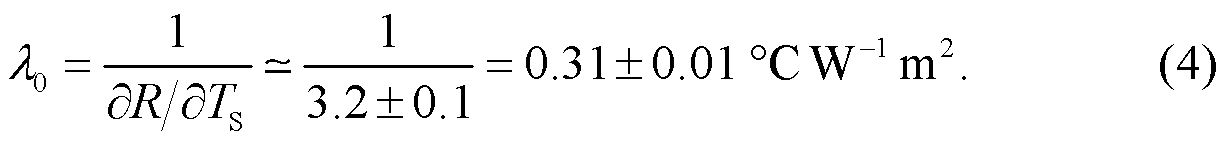The second partial derivative, of OLR with respect to the logarithm of the CO2 concentration, is given by spectroscopy, based on radiative properties of CO2 found in the laboratory. It is calculated from CO2’s many absorption lines, under the temperatures and pressures found in the atmosphere. If the CO2 concentration doubles then ΔL is one, so this partial derivative (after switching signs) is the decrease in OLR per doubling of the CO2 concentration when everything else is held constant, and its value is reported in AR5 (p. 8SM-7) asAgain, we accept this figure. People we know have checked it carefully and accept it, and it is replicable from laboratory physics.

With these values of the partial derivatives, Eq. (3) now fully answers the question about how much OLR changes in our hypothetical situation.

### The Balance between Temperature and CO2

To convert the description of how OLR changes in Eq.s (3), (4), and (5) into a description of a balance between decreasing OLR due to more CO2 and increasing OLR due to warming, let’s consider a move between two steady states in our hypothetical situation where only CO2 and temperature change. Assuming the planet stays close to steady state as CO2 increases, the incremental changes dX become small changes ΔX, so Eq. (3) becomeswhere we have also substituted in the values of the partial derivatives from Eq.s (4) and (5).

The description of the balance then arises from noting that the ASR A is independent of the surface temperature and the CO2 level L, so there is no change in ASR — holding everything else constant breaks any indirect linkages from TS and L to A. But the change in OLR is equal to the change in ASR between steady states (Eq. (1)), so ΔR must also be zero — that is, the decrease in OLR due to extra CO2 is exactly balanced by the increase in OLR due to tropospheric warming.

Noting that ΔR is zero in Eq. (6) gives our balance (after a little rearranging):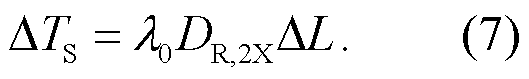This says that the surface warming is proportional to the logarithm of the change in CO2 concentration (which we already knew, right?), but more importantly it also tells us the proportionality constant—how much surface warming there is for a given change in CO2.

### Interpretation

In this situation, the doubling of CO2 can be viewed as a two-stage process:

1. CO2 doubles, holding everything else constant, which reduces OLR by DR,2X.
2. Then the troposphere warms, holding everything else constant, exactly enough to restore the OLR back to its original level.

Or the two stages can be considered as two processes that occur simultaneously without interfering with each other and we add the OLR changes they each cause (the climate is linear for small changes, so processes and changes superpose).

### Diagram

For extra clarity about connections and operations, in this blog series we make liberal use of diagrams of computations — which provide more insight than bunches of simultaneous equations, as long known by those dealing with electrical circuits. In these diagrams arrows indicate the direction of computation or information flow. Fig. 2 illustrates the analysis of this situation, with Eq. (7) providing the computational path.Figure 2: Conventional basic climate model, for the hypothetical situation where only temperature and CO2 can vary.

### No-Feedbacks Sensitivity

By Eq. (7) the warming per doubling of CO2 when everything else is held constant, called the “no-feedbacks equilibrium climate sensitivity”, is the warming when the base-2 logarithm of the CO2 concentration increases by one, namelyThis is the conventional answer. ~1.2 °C is the answer to the question: how much would the surface of the Earth warm if the CO2 concentration doubled, if only the CO2 concentration and the tropospheric temperatures could change and all the tropospheric temperatures changed by the same amount?

It’s a long way from finding the sensitivity of the Earth to an increased concentration of CO2, but it’s a start.

### References

[1^] Held, I. M., & Soden, B. J. (2000). Water Vapor Feedback and Global Warming. Annu. Rev. Energy. Environ., 25:441–75. http://www.dgf.uchile.cl/~ronda/GF3004/helandsod00.pdf

[2^] Pierrehumbert, R. T. (2010). Principles of Planetary Climate. Cambridge: Cambridge University Press. This seems to be legit: http://cips.berkeley.edu/events/rocky-planets-class09/ClimateVol1.pdf. See section 3.4.2, pages 135 – 138 of this pdf.

[3^] IPCC. (2013). Fifth Assessment Report. Cambridge University Press.

VN:F [1.9.22_1171]